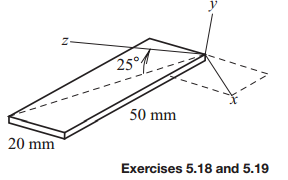### Create an Account

Home / Questions / The x axis lies in the plane of the 500 g plate and the y axis is elevated at 25◦ above th...

# The x axis lies in the plane of the 500 g plate and the y axis is elevated at 25◦ above the diagonal Determine the inertia matrix of the plate relative to the xyz coordinate system in the sketch

The x axis lies in the plane of the 500-g plate, and the y axis is elevated at 25◦ above the diagonal. Determine the inertia matrix of the plate relative to the xyz coordinate system in the sketch.Jun 16 2020 View more View LessSubscribe To Get Solution